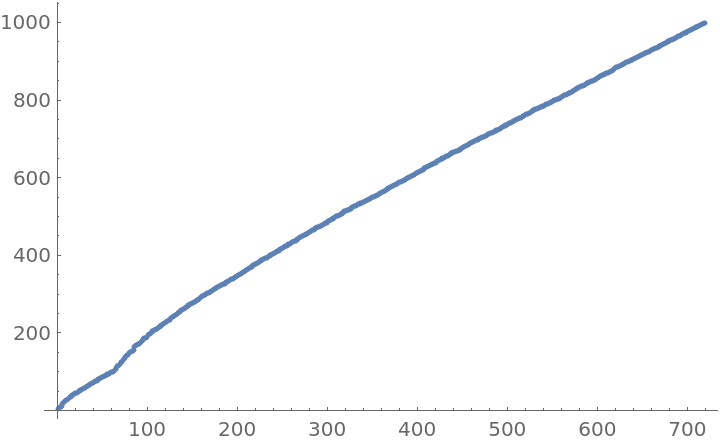Function Repository Resource:

WastefulNumberQ

Test whether an integer is a wasteful number

Contributed by: Sander Huisman
 ResourceFunction["WastefulNumberQ"][n] gives True if the number of digits in the prime factorization of n (including powers) uses more digits than the number of digits of n.

Details and Options

A number is said to be wasteful if the digits used in the prime factorization of a number n (including the number used for the powers) uses more digits than the original number n.
ResourceFunction["WastefulNumberQ"] has the attribute Listable.
The powers for numbers that are raised to the power of 1 are not counted (e.g. 71=7).
For negative numbers, the minus is ignored.

Examples

Basic Examples

Test whether 8 is a wasteful number:

 In:=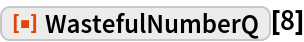Out=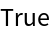Test whether 10 is a wasteful number:

 In:=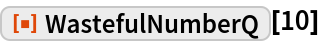Out=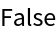Scope

Test multiple digits at once:

 In:=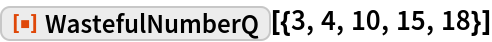Out=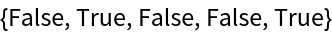Test a negative number:

 In:=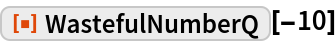Out=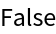Properties and Relations

For negative numbers, the minus is ignored:

 In:=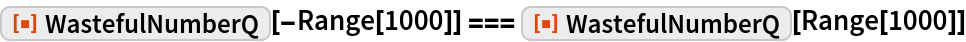Out=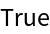Neat Examples

Find all the wasteful numbers up to 1000 and plot them:

 In:=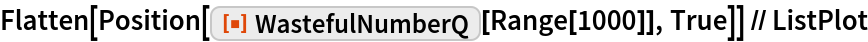Out=